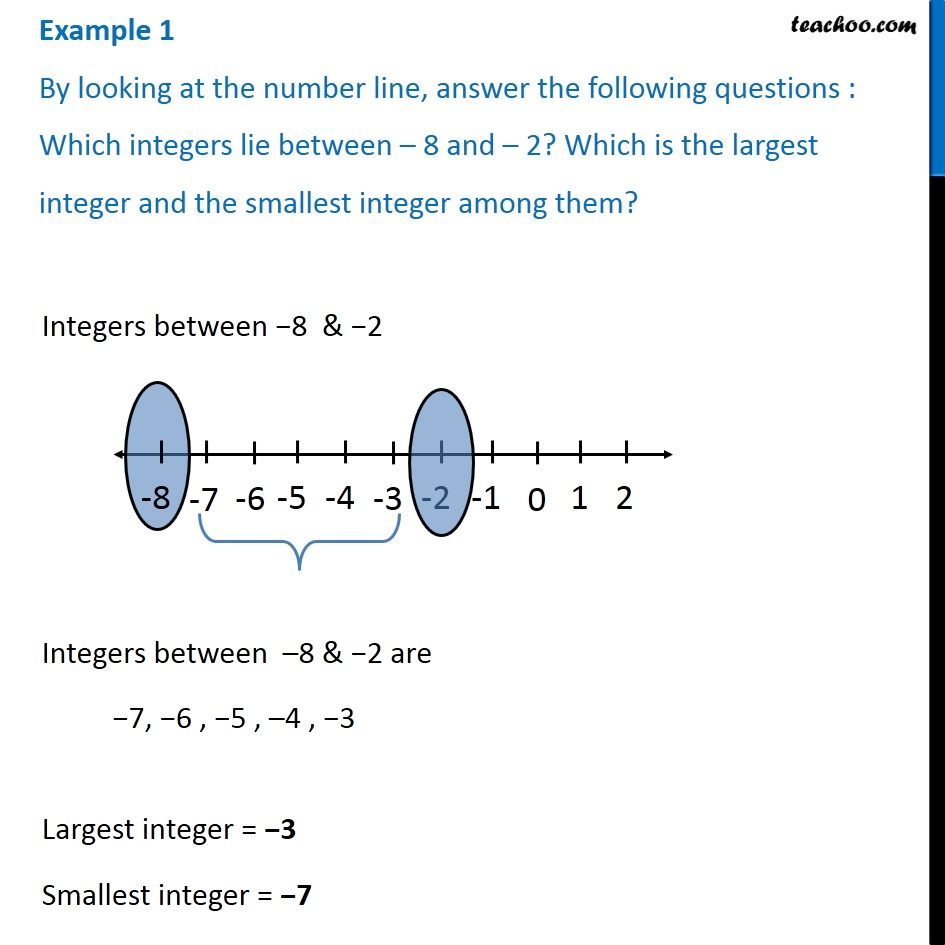1. Chapter 6 Class 6 Integers
2. Serial order wise
3. Examples

Transcript

Example 1 By looking at the number line, answer the following questions : Which integers lie between – 8 and – 2? Which is the largest integer and the smallest integer among them? Integers between −8 & −2 Integers between –8 & −2 are −7, −6 , −5 , –4 , −3 Largest integer = −3 Smallest integer = −7